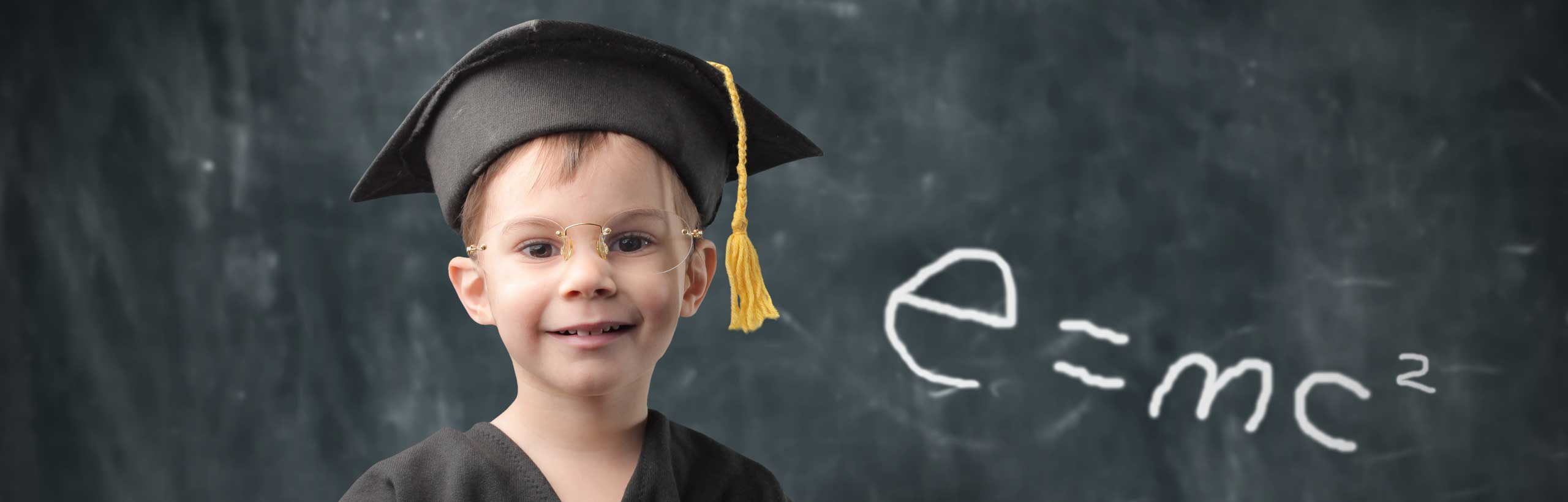# Solve Second Order Differential Equations - part 1

A tutorial on how to solve second order differential equations with auxiliary equation having 2 distinct real solutions. Examples with detailed solutions are included.

 Example 1: Solve the second order differential equation given by y" + 2 y' - 3 y = 0 Solution to Example 1 The auxiliary equation is given by k2 + 2 k - 3 = 0 Factor the above quadratic equation and solve for k (k + 3)(k - 1) = 0 k1 = -3 and k2 = 1 The general solution to the given differential equation is given by y = A ek1 x + B ek2 x = A e-3 x + B ex where A and B are constants. Example 2: Solve the second order differential equation given by y" + 3 y' -10 y = 0 with the initial conditions y(0) = 1 and y'(0) = 0 Solution to Example 2 The auxiliary equation is given by k2 + 3 k - 10 = 0 Solve the above quadratic equation to obtain k1 = 2 and k2 = - 5 The general solution to the given differential equation is given by y = A e2 x + B e- 5 x where A and B are constants that may be evaluated using the initial conditions. y(0) = 1 gives y(0) = A e0 + B e0 = A + B = 1 y'(0) = 0 gives y'(0) = 2 A e0 - 5 B e0 = 2 A - 5 B = 0 Solve the system of equations A + B = 1 and 2 A - 5 B = 0 to obtain A = 5 / 7 and B = 2 / 7 The solution to the given equation may be written as y = (5 / 7) e2 x + (2 / 7) e- 5 x Exercises: Solve the following differential equations. 1. y" + 5 y' - 6 y = 0 2. y" + y' - 2 y = 0 with the initial conditions y(0) = 2 and y'(0) = 0 Answers to Above Exercises 1. y = A e x + B e -6 x , A and B constant 2. y = (4 / 3) e x + (2 / 3) e -2 x More references on Differential Equations Differential Equations - Runge Kutta Method